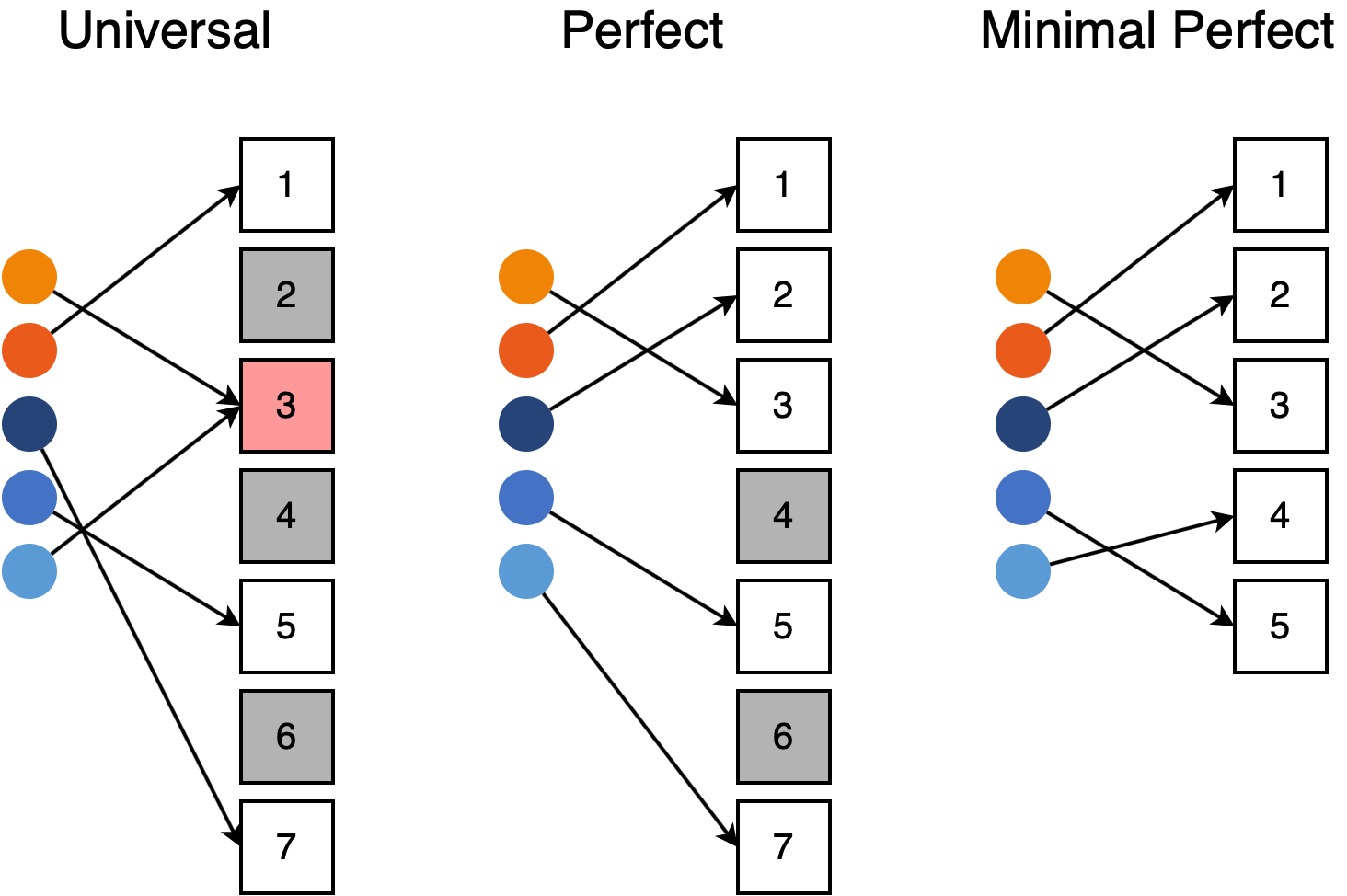# Minimal perfect hash functions

Posted by Ben Coleman on September 12, 2019 · 11 mins read

If you want to observe a hash function in the wild, all you need to do is look under the hood of the nearest key-value store. Hashing is a fundamentally simple idea with an enormous scope; hash-based data structures are present nearly everywhere in practice. Most programmers are familiar with random hash functions. However, there is an incredibly diverse taxonomy of different hashes, including more exotic constructions such as cryptographic hashes and locality sensitive hash functions. In this post, we provide a user-friendly introduction to the literature on minimal perfect hash functions.

### What is a minimal perfect hash?

At a high level, minimal perfect hash functions use information about the input to avoid collisions and improve resource utilization. To illustrate what makes a hash function minimal and perfect, suppose we construct a hash table for N distinct key-value pairs. When we apply a universal hash with range [1,R] to our set of keys, several of the keys will collide, or have same hash value, even when R > N. To distinguish these keys in the table, we have to resolve the collisions using chaining, probing or other addressing schemes. A perfect hash function is one that maps N keys to the range [1,R] without having any collisions. A minimal perfect hash function has a range of [1,N]. We say that the hash is minimal because it outputs the minimum range possible. The hash is perfect because we do not have to resolve any collisions.Here, R = 7 and N = 5. The universal hash has unused buckets and collisions. The perfect hash has no collisions, and the MPH has neither collisions nor unused buckets.

### Why do you want a minimal perfect hash?

Tables constructed using minimal perfect hash (MPH) functions tend to be smaller than normal hash tables because there are no empty memory locations in the table. Table lookups are also faster because we do not need collision resolution, leading to an absolute worst-case O(1) lookup. Best of all, the most compact MPH functions only require 2.07 bits per key. Since ordinary hash tables need to store the keys explicitly, this can net an enormous memory savings. Thanks to lots of research from the theory community, MPH functions are not terribly expensive to construct. We have methods that can scale to more than 1 billion keys.

However, there are a couple of downsides. Unfortunately, we cannot insert new entries without regenerating the entire table. We need to know all of the keys in advance. Worse, if we discard the original keys to save space, we cannot say whether a given key was part of our original key set. Under these conditions, attempting to look up a key that was not present in our original set will return a random element from the table. While this issue can be mitigated by storing hash codes of the elements, the disadvantages mean that MPH tables are not a drop-in replacement for hash tables.

MPH functions are mainly useful for read-only caches and as a way to implement random bijective maps for other algorithms. But they do this really well - in practice, the O(1) lookup time can yield a 10x speedup for in-memory cache lookups. For on-disk data, MPH functions only require one disk access.

### How do we construct a minimal perfect hash?

To generate a MPH, we start with a collection of non-minimal imperfect hash functions. The intuition is that if we apply enough hashes to two keys, at least one pair of hash functions will not collide. Most MPH algorithms index into a lookup table $$g$$ with several universal hashes. The output is a function of the lookup table values. These values are chosen so that the resulting function is both minimal and perfect.The figure shows an example where we use three universal hashes and output the sum of the lookup table values.

### Linear Algebra

To see how we might go about creating an MPH, let’s view the problem as a linear system and solve for the lookup table. Create a matrix $$H$$ where each row corresponds to a key and each column corresponds to a hash value. We will place a 1 in $$H[i,j]$$ if one of our hash functions maps key $$i$$ to value $$j$$ and 0 otherwise.If we take the matrix-vector product of $$H$$ with $$g$$, we get MPH values. Note that this system is underdetermined when the range of our hashes $$R > N$$. Even with random initializations of $$H$$, the system is still solvable with high probability. I generated the example MPH by explicitly solving for $$g$$, but we really do not want to solve this system in practice. Without applying tricks, this operation is worse than $$O(N^2)$$ which is prohibitive for large $$N$$.

### Hypergraph Peeling

Many MPH algorithms get around this problem using hypergraph peeling, which is a simple message passing algorithm on hypergraph edges. We start by constructing a hypergraph from the hash values and keys. Each hash value is a node and each key defines an edge of the hypergraph. Assuming the hypergraph is peelable, we can find the lookup table in linear time. To illustrate, here is the hypergraph corresponding to our example.To peel a hypergraph, we start by finding an edge that has at least one node that it does not share with other edges. Then, we remove or peel this edge from the graph and repeat the process. When we remove an edge, other edges which were not previously peelable may become peelable. Informally, the entire hypergraph is peelable if we can remove every edge using this process. The output of this process is a list of edges in the peeling order. Finding the lookup table only requires one pass through this list.

A detailed discussion of how to get the lookup table from the edge list is beyond the scope of this blog post. We refer the interested reader to the algorithm in  and conclude with an interesting observation. If we examine the hypergraph MPH literature, we find that the overwhelming majority of algorithms use three random hash functions in practice. It turns out that this is not an arbitrary choice. When we use three hashes, the resulting hypergraph is peelable with much higher probability than when we only use two.

### More methods

So far, we have presented two ways to view the MPH construction problem: linear systems and random graphs. These are the two most illustrative ways to look at the problem, but the MPH literature is much more diverse. For instance, we can store binary values that decide whether the hash function in question had a collision . We can directly solve the linear system using modern linear algebra tricks  or employ a diverse selection of methods to analyze the random graphs [1,4,5]. Other methods rely on adding integer displacements to resolve collisions [6,7]. If we extend our discussion to perfect hash functions, we have an even wider array of candidates including cuckoo hashing  and the methods used by gperf .

### Concluding Remarks

Minimal perfect hash functions are good building blocks for other algorithms and database systems. In this post, we only talked about read-only hash tables and caching, but MPH functions have many other uses. Space-efficient bijective maps are generally a useful primitive and this is exactly the intended use case for an MPH function. We also introduced two ways to view the MPH problem and briefly discussed a variety of other approaches.

 Botelho, F. C., Pagh, R., & Ziviani, N. (2007, August). Simple and space-efficient minimal perfect hash functions. In Workshop on Algorithms and Data Structures (pp. 139-150). Springer, Berlin, Heidelberg.

 Limasset, A., Rizk, G., Chikhi, R., & Peterlongo, P. (2017). Fast and scalable minimal perfect hashing for massive key sets. arXiv preprint arXiv:1702.03154.

 Genuzio, M., Ottaviano, G., & Vigna, S. (2016, June). Fast scalable construction of (minimal perfect hash) functions. In International Symposium on Experimental Algorithms (pp. 339-352). Springer, Cham.

 Czech, Z. J., Havas, G., & Majewski, B. S. (1992). An optimal algorithm for generating minimal perfect hash functions. Information processing letters, 43(5), 257-264.

 Botelho, F. C., Kohayakawa, Y., & Ziviani, N. (2005, May). A practical minimal perfect hashing method. In International Workshop on Experimental and Efficient Algorithms (pp. 488-500). Springer, Berlin, Heidelberg.

 Pagh, R. (1999, August). Hash and displace: Efficient evaluation of minimal perfect hash functions. In Workshop on Algorithms and Data Structures (pp. 49-54). Springer, Berlin, Heidelberg.

 Belazzougui, D., Botelho, F. C., & Dietzfelbinger, M. (2009, September). Hash, displace, and compress. In European Symposium on Algorithms (pp. 682-693). Springer, Berlin, Heidelberg.

 Pagh, R., & Rodler, F. F. (2004). Cuckoo hashing. Journal of Algorithms, 51(2), 122-144.

 Schmidt, D. C. (2000). Gperf: A perfect hash function generator. More C++ gems, 461-491.